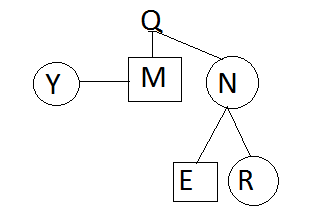Gurpurab Offer - Use Code GURPURAB2023

# Blood Relation Quiz With Solution For IBPS PO: Part 3

#### Direction:

• A\$B means A is sister of B
• A@B means A is father of B
• A>B means A is wife of B
• A<B means A is son of B
• A=B means A is mother of B

1. Which of the following expressions means ‘P’ is daughter of ‘S’?
a) P<Q>S@R
b) S=Q>P<R
c) S>R@P\$Q
d) P@S>R<Q
e) R@Q\$S=P

2. How is ‘Q’ related to ‘R’?
P@Q=S>R<T
a) Mother
b) Son
c) Father
d) Father in law
e) Mother in law

3. How is ‘T’ related to ‘V’?
X\$Y@V<U\$T
a) Uncle
b) Aunt
c) Cousin sister
d) son
e) C.N.D.

4. M is the son of Q. N, M’s sister has a daughter R and a son E. Y is maternal aunt of R.
How is Y related to N? If N has only one brother.
a) Brother in law
b) Sister
c) Mother
d) Sister in law
e) Aunt

5. P is son Q. Q is mother of V. V is sister of U. U is father of X. Y is daughter of P. V is wife of N.
How is ‘Y’ related to ‘x’?
a) Sister
b) Brother
c) Cousin sister
d) Aunt
e) Mother

#### 1. Ans. C.

Solution:#### 2. Ans. E.

Solution:#### 3. Ans. E.

Solution:#### 4. Ans. D.

Solution:#### 5. Ans. C.

Solution: